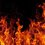# Physics vs. Maths

From a long old original story of the tortoise and the hare which I think all must have heard in which there is a race between the hare and the tortoise , hare sleeps, tortoise wins....... now in the original story it was given that the speed of tortoise is 1/10th of that of hare and also the hare was 1000 m behind the tortoise. Let us now assume there is no finish line, they can run as much as they like. Fare enough . What our common sense says there would definately be one point where they will meet , good , physics agrees will your common sense . Now, to find the distance when they meet we'll make a equation . As Let x be the speed of hare and t be the time they meet . We find they would meet at 1000/9 m from the place where the hare started. Enough of physics now let's play some maths here. Since the speed of the tortoise is 1/10 th of hare, so when hare covers 1000 m the tortoise would have covered 100 m more , when the hare covers that 100 m , the tortoise would still be ahead by 10 m , when this 10 m are covered by the hare the tortoise is still ahead by 1 m , when this 1 m is covered the tortoise would still manage 0.1 m and so on.....So realistically they would never meet , the tortoise would always be ahead by some distance although very very small. How come there is so difference in opinions of maths and physics ?Note by Utkarsh Dwivedi
6 years, 4 months ago

This discussion board is a place to discuss our Daily Challenges and the math and science related to those challenges. Explanations are more than just a solution — they should explain the steps and thinking strategies that you used to obtain the solution. Comments should further the discussion of math and science.

When posting on Brilliant:

• Use the emojis to react to an explanation, whether you're congratulating a job well done , or just really confused .
• Ask specific questions about the challenge or the steps in somebody's explanation. Well-posed questions can add a lot to the discussion, but posting "I don't understand!" doesn't help anyone.
• Try to contribute something new to the discussion, whether it is an extension, generalization or other idea related to the challenge.

MarkdownAppears as
*italics* or _italics_ italics
**bold** or __bold__ bold
- bulleted- list
• bulleted
• list
1. numbered2. list
1. numbered
2. list
Note: you must add a full line of space before and after lists for them to show up correctly
paragraph 1paragraph 2

paragraph 1

paragraph 2

[example link](https://brilliant.org)example link
> This is a quote
This is a quote
    # I indented these lines
# 4 spaces, and now they show
# up as a code block.

print "hello world"
# I indented these lines
# 4 spaces, and now they show
# up as a code block.

print "hello world"
MathAppears as
Remember to wrap math in $$ ... $$ or $ ... $ to ensure proper formatting.
2 \times 3 $2 \times 3$
2^{34} $2^{34}$
a_{i-1} $a_{i-1}$
\frac{2}{3} $\frac{2}{3}$
\sqrt{2} $\sqrt{2}$
\sum_{i=1}^3 $\sum_{i=1}^3$
\sin \theta $\sin \theta$
\boxed{123} $\boxed{123}$